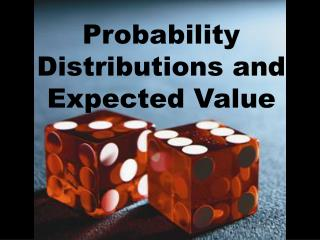Download PresentationProbability Distributions and Expected Value

# Probability Distributions and Expected Value

Download Presentation## Probability Distributions and Expected Value

- - - - - - - - - - - - - - - - - - - - - - - - - - - E N D - - - - - - - - - - - - - - - - - - - - - - - - - - -
##### Presentation Transcript

1. Probability Distributions and Expected Value

2. In previous chapters, our emphasis was on the probability of individual outcomes. • This chapter develops models for distributions that show the probabilities for all possible outcomes of an experiment.

3. Random Variable (X) • Has a single value, x, for each outcome of an experiment. To show all the possible outcomes, a chart is normally used.

4. Discrete variables take values that are separate (or that can be “counted”)Continuous variables have an infinite number of possible outcomes (usually measurements that can have an unlimited decimal place)

5. For example: • The number of phone calls made by a salesperson • Discrete (1,2,3,4,5…..)

6. For example: • The length of time a person spends on the phone • Continuous (1 min, 1.23min …..)

7. Let a DRV (X) be the possible outcomes when rolling a die The probability distribution could be written in a table This is a uniform distribution, because all the probabilities are the same.

8. A graph would look like this… 1 1/6 2 3 4 5 6

9. Expected Value: Informal When rolling 2 dice, the sum that is generated most frequently is called the expected value. (7) This can also be calculated mathematically. Multiply each roll by it’s probability of occuring…

10. E(sum) = 2P(sum = 2) + 3P(sum = 3) + … +…12P(sum = 12) = 2 X 1 / 36 + 3 X 2 / 36 … = 252 / 36 = 7

11. The expected value, E(X), is the predicted average of all possible outcomes.It is equal to the sum of the products of each outcome with its probability

12. n = xiP(X = xi) i = 1 Expected Value of a Discrete Random Variable The sum of the terms of the form (X)(P[X]) E(X) = x1P(X = x1) + x2P(X = x2) + … + xnP(X = xn)

13. Ex 1: Suppose you toss 3 coins. • What is the likelihood that you would observe at least two heads? • What is the expected number of heads?

14. 1 3 3 1 8 8 8 8 Represent the theoretical probability distribution as a table.The DRV, X, represents the number of heads observed. X 0 hs 1 hs 2 hs 3 hs P(X) = x

15. a) P(X = 2) + P(X = 3) = 3 / 8 + 1 / 8 = 1 / 2 b) The expected number of heads = 0(1 / 8) + 1(3 / 8) + 2(3 / 8) + 3(1 / 8) = 3 / 2

16. For a game to be fair, E(X) must be zero Consider a dice game If you roll a 1 2 3 you win \$1.00 If you roll a 4 5 6 you pay \$1.00 Is this game fair? E(X) = (1)(1/6) + (1)(1/6) + (1)(1/6) + (-1)(1/6) + (-1)(1/6) + (-1)(1/6)

17. Page 374 1, 2(ex 2), 3a,c, 4, 9, 11,12, 19